# Archive for the ‘Math’ Category:

### The Mona Lisa As Drawn By A Traveling Salesman

Posted In: Fun | Math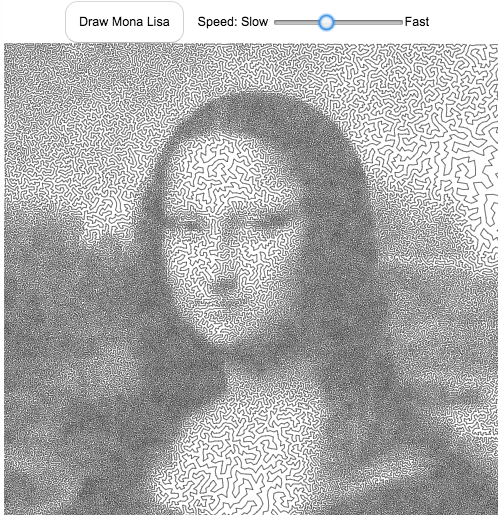The traveling salesman problem (TSP) is a classic problem in operations research and mathematical programming. It seeks to answer the question, given a set of destinations, what is the shortest possible travel route someone could take to visit them all. It is a notoriously challenging problem to solve when the number of destinations grows. A picture of the famous Mona Lisa was converted to 100,000 dots and a challenge was created to find the most optimal solution to visiting each of these 100 thousand points (i.e. drawing the shortest continuous line that goes through each and every point.
The current most-optimal solution that has been found to this Mona Lisa challenge was calculated in 2009 by Yuichi Nagata. This art is created using the order of destinations (also called the “tour) that he developed.

Click the “Draw Mona Lisa” button below to see the famous painting drawn with a single continuous line with 100,000 segments.

Each time you click on the “Draw Mona Lisa” button, it chooses a different starting point but follows the same order of destination points, looping around until all the points are visited.

You can also change the speed at which the individual line segments are drawn. It is surprisingly relaxing and satisfying to watch the line wind its way around the screen to fill in the famous picture of the Mona Lisa.

For a very high res version of the entire photo click here (around 4 MB file size).

Data and Tools:
I downloaded the 100,000 points and optimal tour from Yuichi Nagata from the TSP Art website and the University of Waterloo.
Javascript was used to draw on the HTML canvas and animate the process.### Estimating pi (π) using Monte Carlo Simulation

Posted In: Math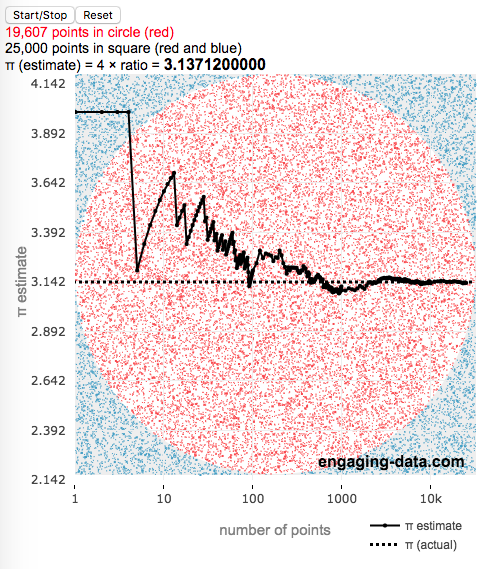This interactive simulation estimates the value of the fundamental constant, pi (π), by drawing lots of random points to estimate the relative areas of a square and an inscribed circle.

Pi, (π), is used in a number of math equations related to circles, including calculating the area, circumference, etc. and is widely used in geometry, trigonometry and physics.

This app estimates the value of pi by comparing the area of a square and an inscribed circle. The areas are calculated by randomly placing dots into the square and then counting how many of them are also inside the circle. If you do this enough times, you will get a rough ratio of the relative areas of the two shapes. These points are plotted on the graph (red if the fall inside the circle and blue if the fall outside).

Also shown on the graph is the value of our estimate of pi as the simulation progresses, from a few points to many thousands, to millions of points. We can see that when we have only a few points, the value may not be very accurate but as the number of points increases the value of our estimate gets closer to the true value. Running the simulation will add and plot 1 million points. After the first 100 points are added, the rate at which points are added increases. You’ll notice this as the speed at which dots fills up the square increases and because the plot is shown with a logarithmic x-axis.

Here is the math:
Length of side of square: $2 \times r$
radius of circle: $r$
Area of square: $A_{square} = 4r^2$
Area of circle: $A_{circle} = \pi r^2$

The ratio of areas is $A_{circle}/A_{square} = \pi r^2 / 4r^2 = \pi / 4$
Solving for pi: $\pi = 4 \times A_{circle}/A_{square} \approx 4 \times N_{dots_{circle}}/N_{dots_{square}}$
So pi is estimated as 4 times the ratio of dots in the circle vs square

Tools:
This was programmed in javascript, canvas and plotted using the open source plotly javascript plotting library.### ¿Cuánto tardarías en contar hasta un millón?

Posted In: Counting | Fun | Math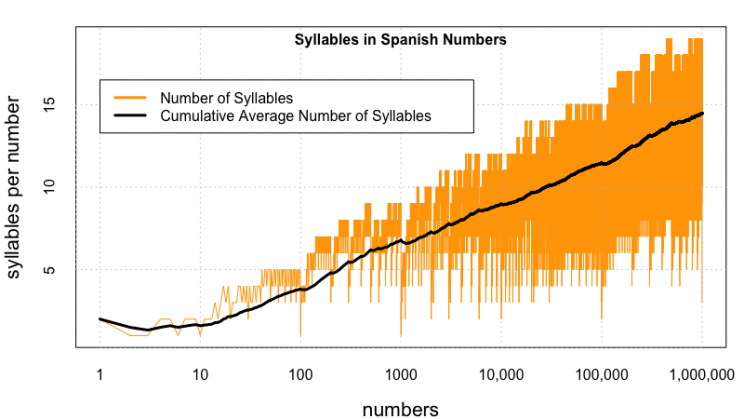Disculpe(n) mi pobre español. Utilicé google translate para escribir esto en español.
Aquí está la calculadora que calculará cuánto tiempo lleva contar un millón (o números mayores) en español.
(more…)

### Counting to One Million in Different Languages (Chinese, English and Spanish)

Posted In: Counting | Math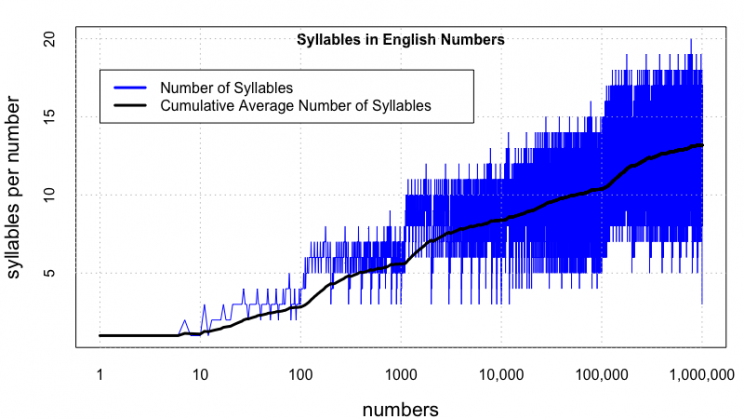Updated: Lots of folks on Reddit pointed out some mistakes in the Spanish calculations, and helped me figure out the solutions, so the Spanish graphs are now updated. The Spanish calculator is now live!

Building off of the last post about Counting to One Million in English, I received some comments about looking at other languages.  That seemed like a very good idea, so I looked at a list of the world’s most popular languages and saw Chinese and Spanish listed with English in the Top 3.  Having a little experience with both of those, I set out to compare how long it’d take to count in each of these languages, if you had to pronounce every single number from one to one million.

Again, here’s the plot of the number of syllables per number for English.  The longest word is seven hundred seventy seven thousand seven hundred seventy seven (20 syllables).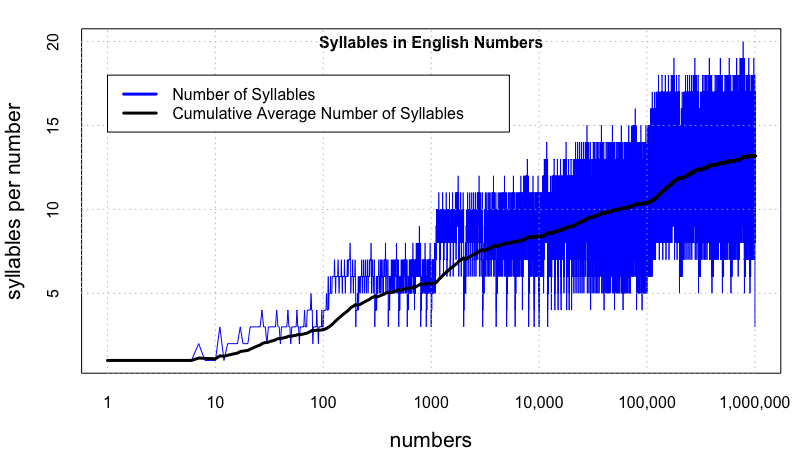(more…)

### How long does it take to count to 1 million? 1 billion? 1 trillion?

Posted In: Math | Programming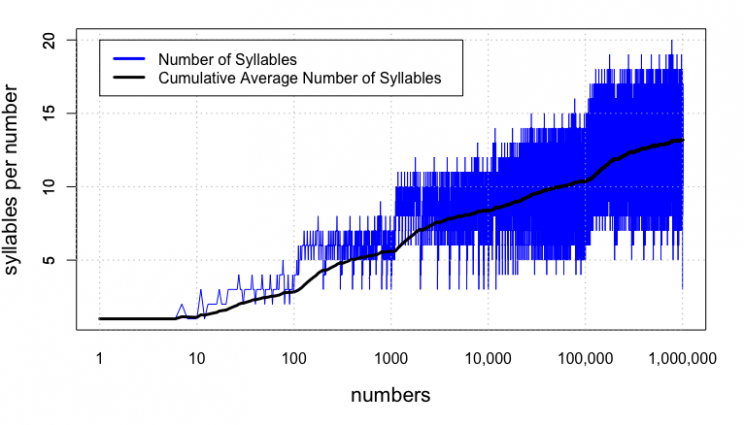My son likes large numbers (like septillion, googol and googolplex) and once asked me how long it would take to count to septillion (which is 1 followed by 24 zeros).  I told him it would take longer than the age of the universe to do that, so he started working his way down.  He asked me about counting to one million.  I did a little math (assuming one number per second) and got about 11-12 days . . . but then thought, the large numbers (like 658,243) take more than a second to say.

Looked on the web a little to see if anyone else had done a more sophisticated calculation.  Lots of calculations were like my own (assuming one number per second).  Others acknowledge that it would take longer for large numbers and made assumptions about what that would be.  But nothing definitive, so I thought I’d make one.  This counting calculator is based on the number of syllables in every number and counts all the syllables you’d have to pronounce in order to count from one to one million (or other numbers).